# Reduction and Approximate Solutions of the Batch Grinding Equation

A principal first order and a minor summation term contribute to the solution of the discretized batch grinding equation when formulated in cumulative retained mode. Depending on the interrelationships between the selection and breakage parameters, the minor term may be advantageously modified in a number of ways.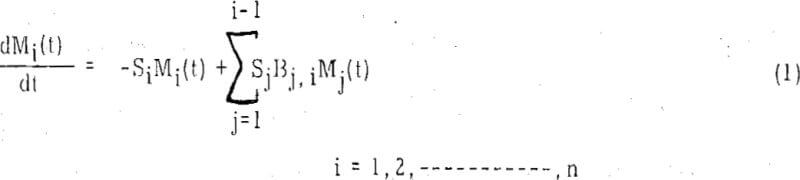where Mi(t) is the mass fraction of particles identified by i-th- size index at time t; Si, the selection function, is the rate of breakage of particles of i-th size, and the breakage function, Bj i, is the i-th size fraction of products when particles of size index j are broken. The practical solution of Equation(1) becomes progressively tedious for i>2. Thus, for i = 3, the solution is

The cumulative fraction of particles retained on i-th size is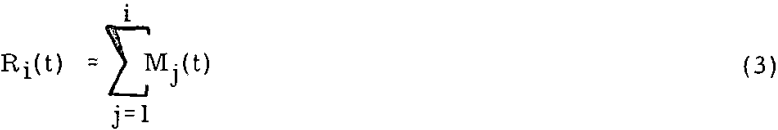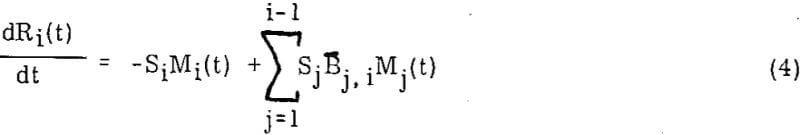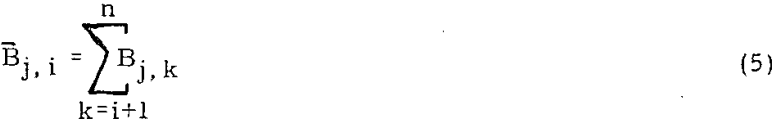Mj(t) = Rj(t) – Rj-i(t)……………………………………………………..(6)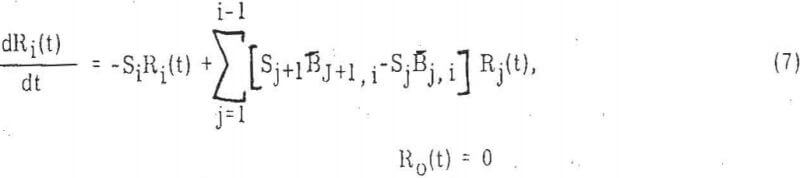Although not strictly necessary for the approximations to be made later on, it is convenient to assume that cumulative breakage function is normalizable which for particles distributed on a mesh scale that varies by a constant ratio becomes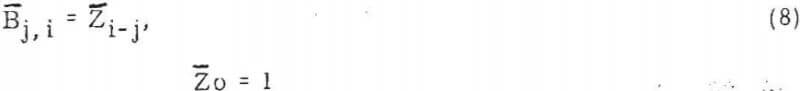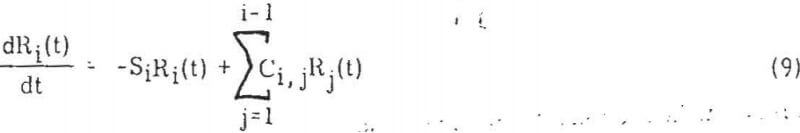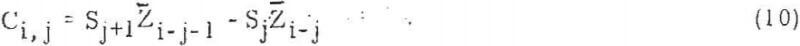A closer examination indicates that Ci, j should be quite small as compared to Si or even sensibly zero. Also, in Equation (9), the principal contribution comes from the first term on the right side, since on physical grounds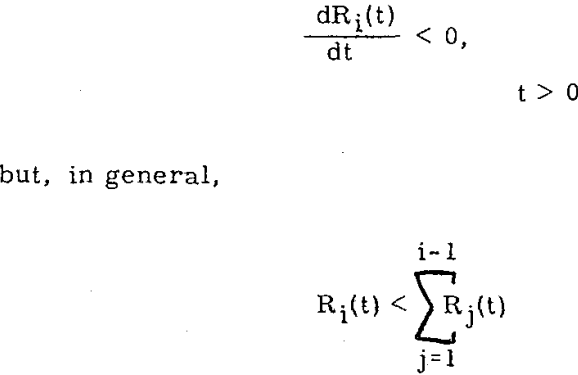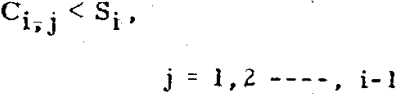Case I: Ci, j → 0

When Ci, j are vanishingly small,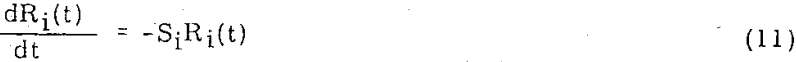whose solution is

Ri(t) = Ri(o) exp [-Sit]…………………………………………………….(12)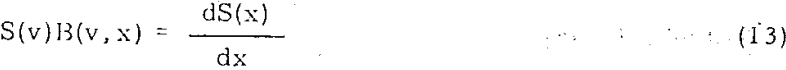where x is particle size, in continuous scale. Now the solution of integro-differential equation of grinding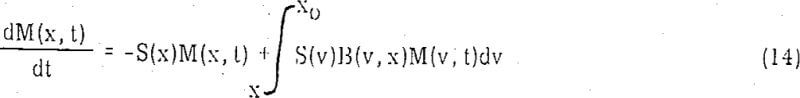R(x, t) – R(x, o) exp [-S(x)t]…………………………………………………(15)

R(x, t) = R(x,o) exp [-S(x)φ(t)]……………………………………………(16)

ln[l-Fi(t)] = -Sit……………………………………………………………..(17)

Fi(t) ≈ Sit……………………………………………………………..(18)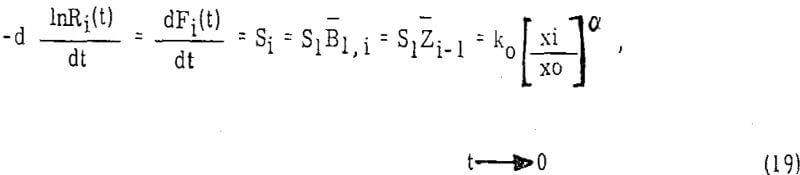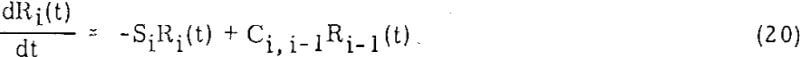For a single size feed at t→ 0, Ri = Ri-1, Equation (20) becomes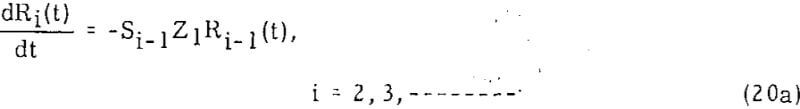The exact practical solution, while perfectly straight-forward, becomes somewhat tedious for higher size indici. On the other hand, since Ci, i-1 is small and the ratio R-1(t)/Ri(t) varies only slowly with time, especially for finer particle sizes, it is possible to derive quite efficiently the approximate solution of Equation (20) by Cauchy-Picard method of successive iterations. Thus, the (n+i)-th approximate solution is given by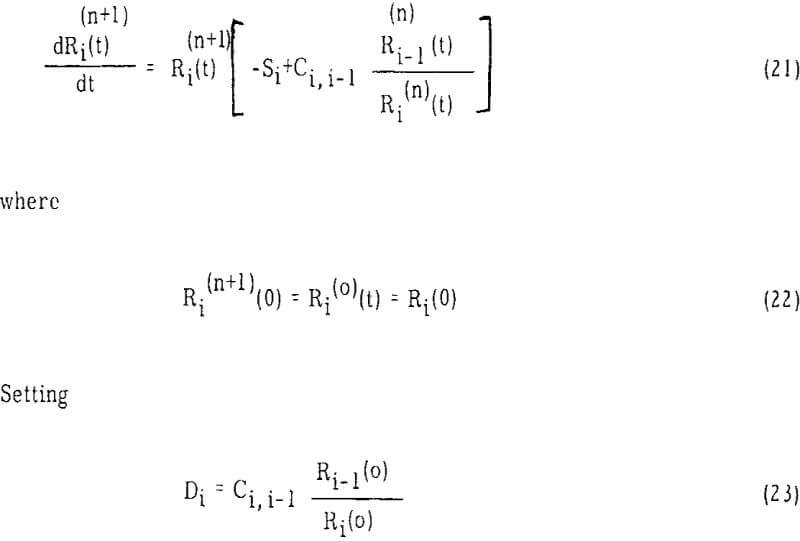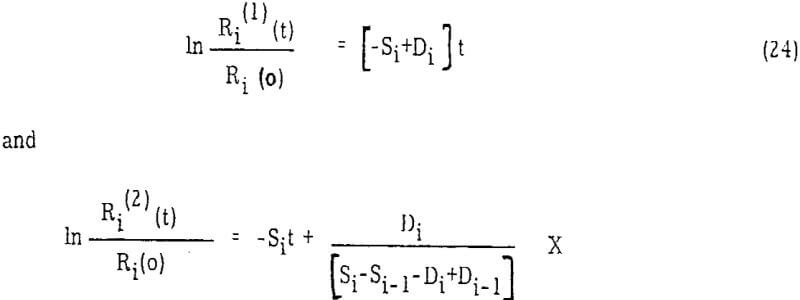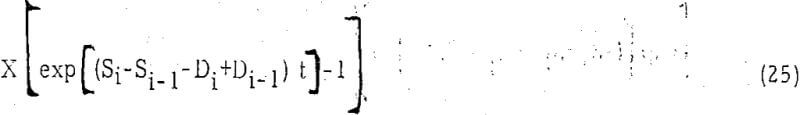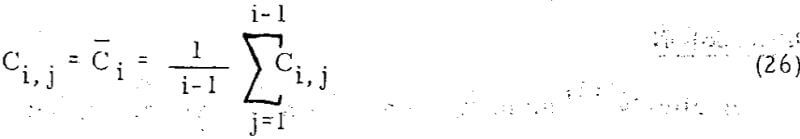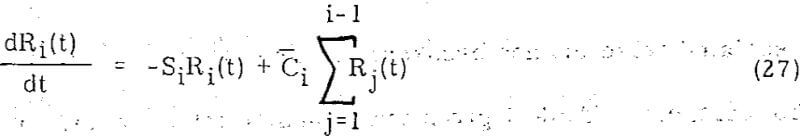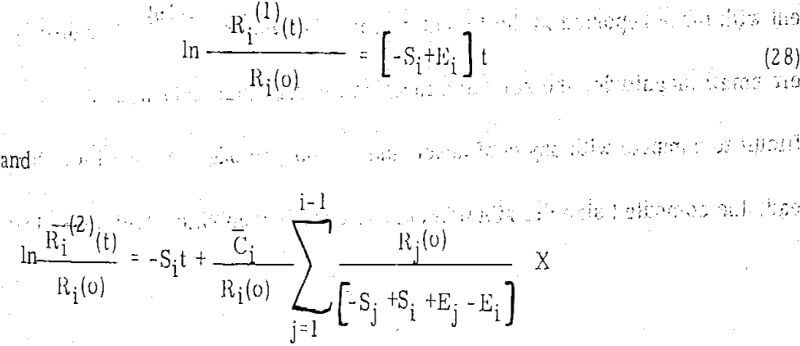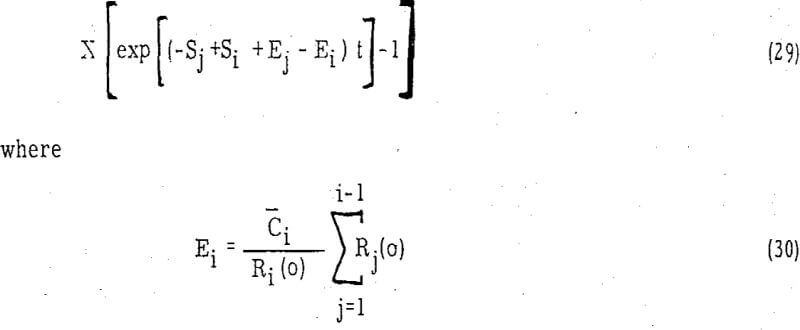Berlioz’s grinding data for single size feed of dolomite dry ground in a batch ball mill is utilized for the purpose of illustrating the approximate solutions. The choice of this data is dictated by the fact that this and a nearly identical comminution system has been extensively studied, and the associated selection and breakage parameters have been computed with reasonable certainty. Next Table gives the values of selection and normalized breakage functions, Ci, i-1 and Ci. The first two functions were computed by this author earlier and were used for simulation of the mill with a high degree of precision.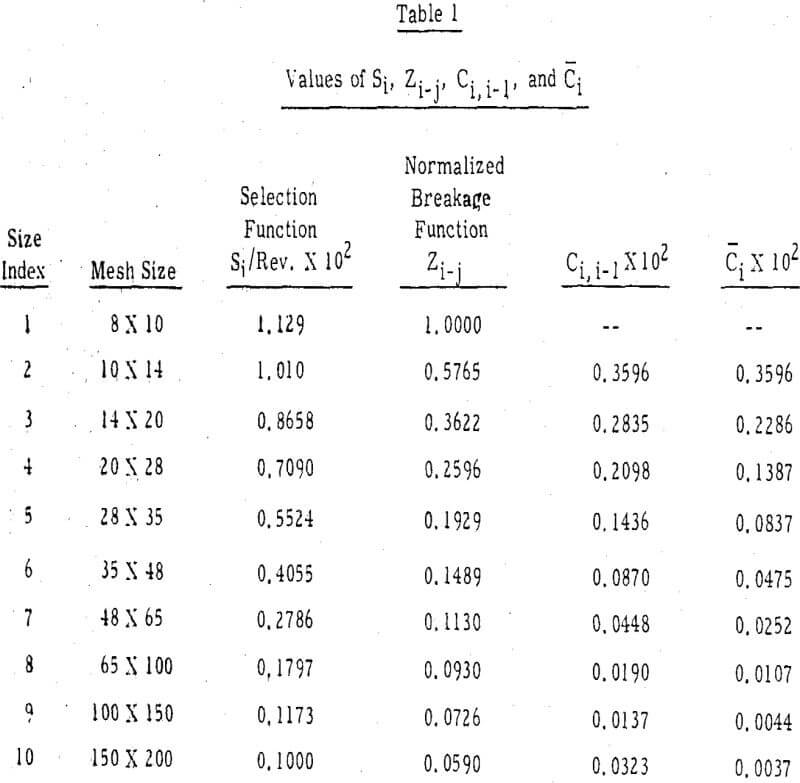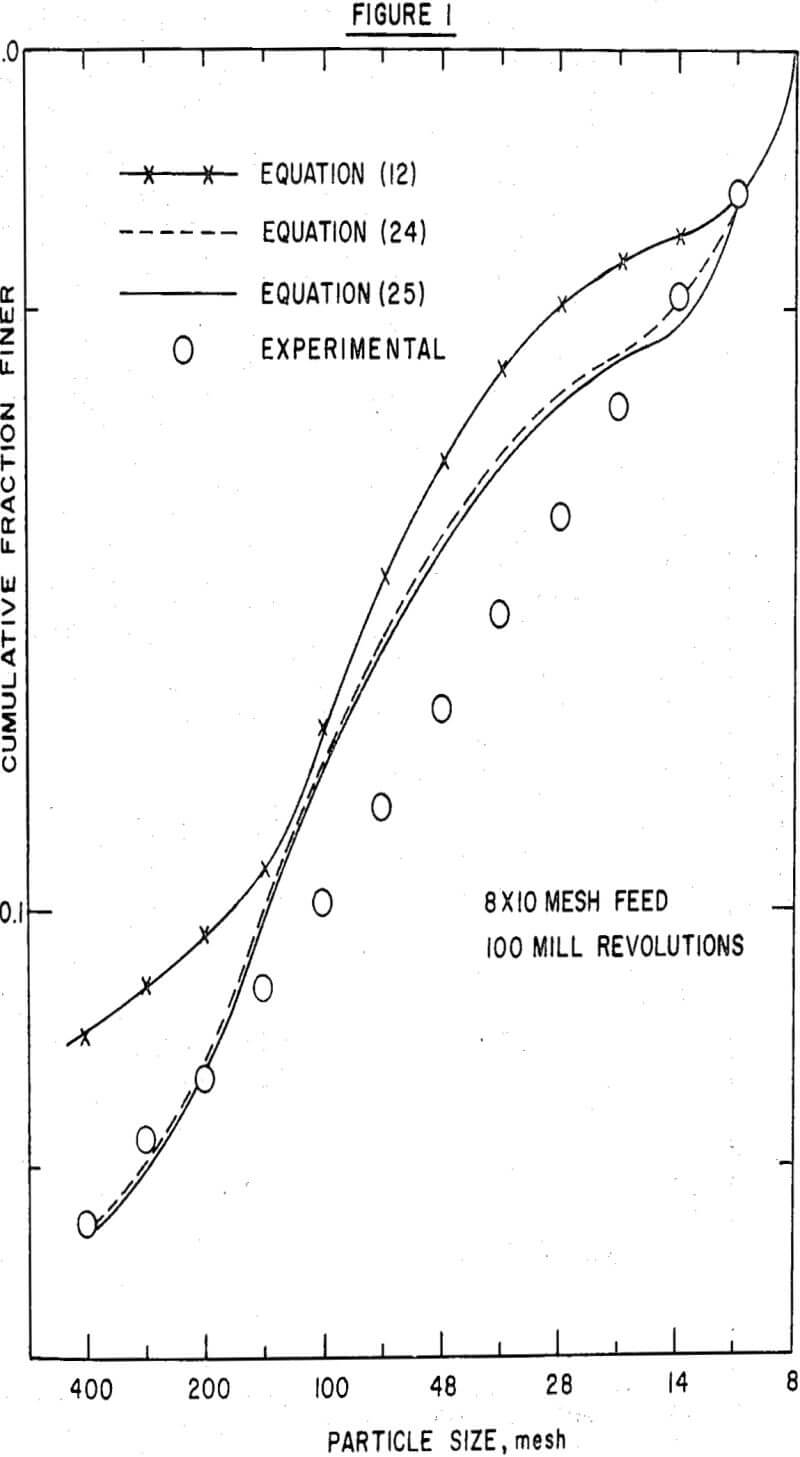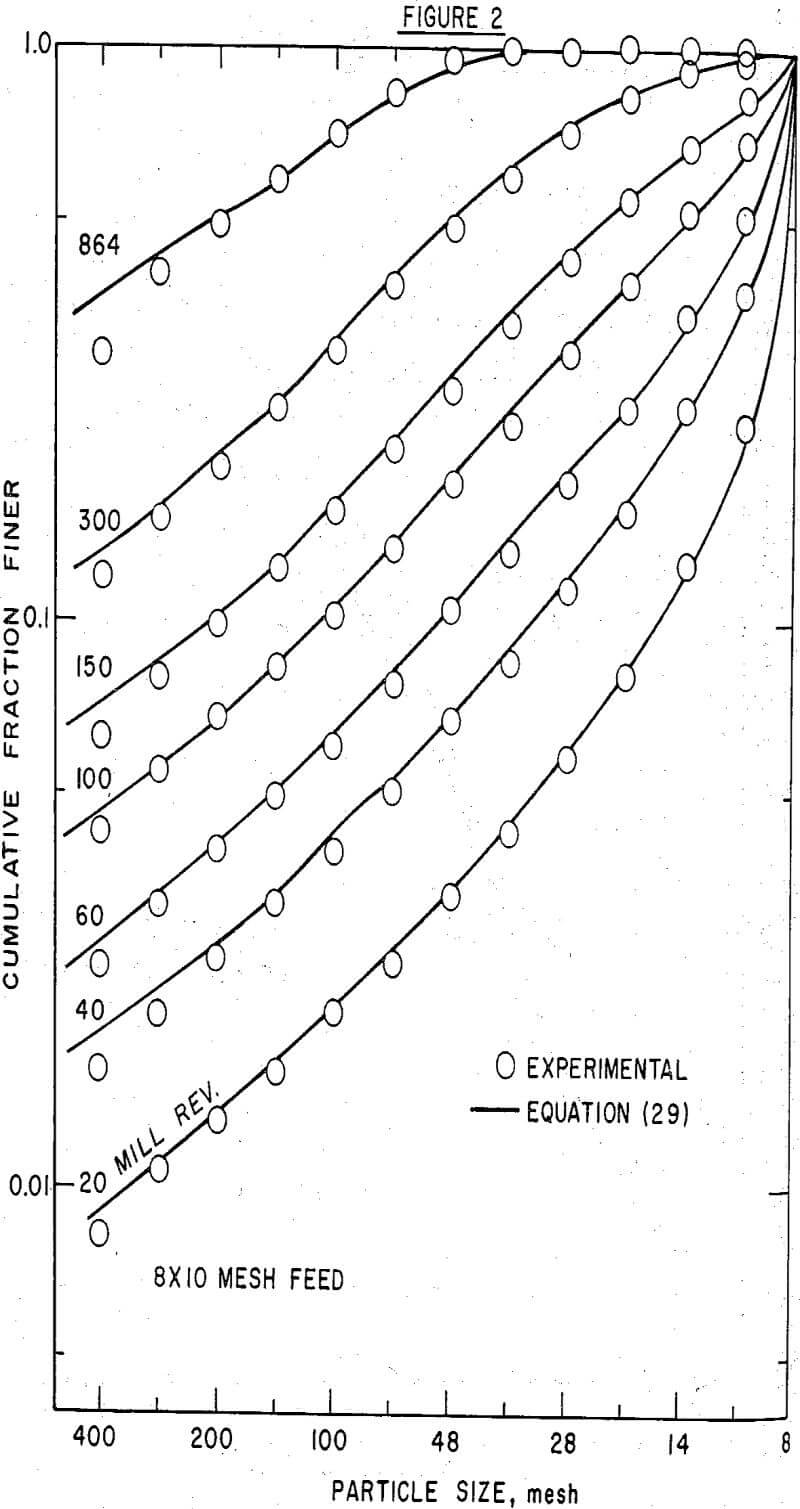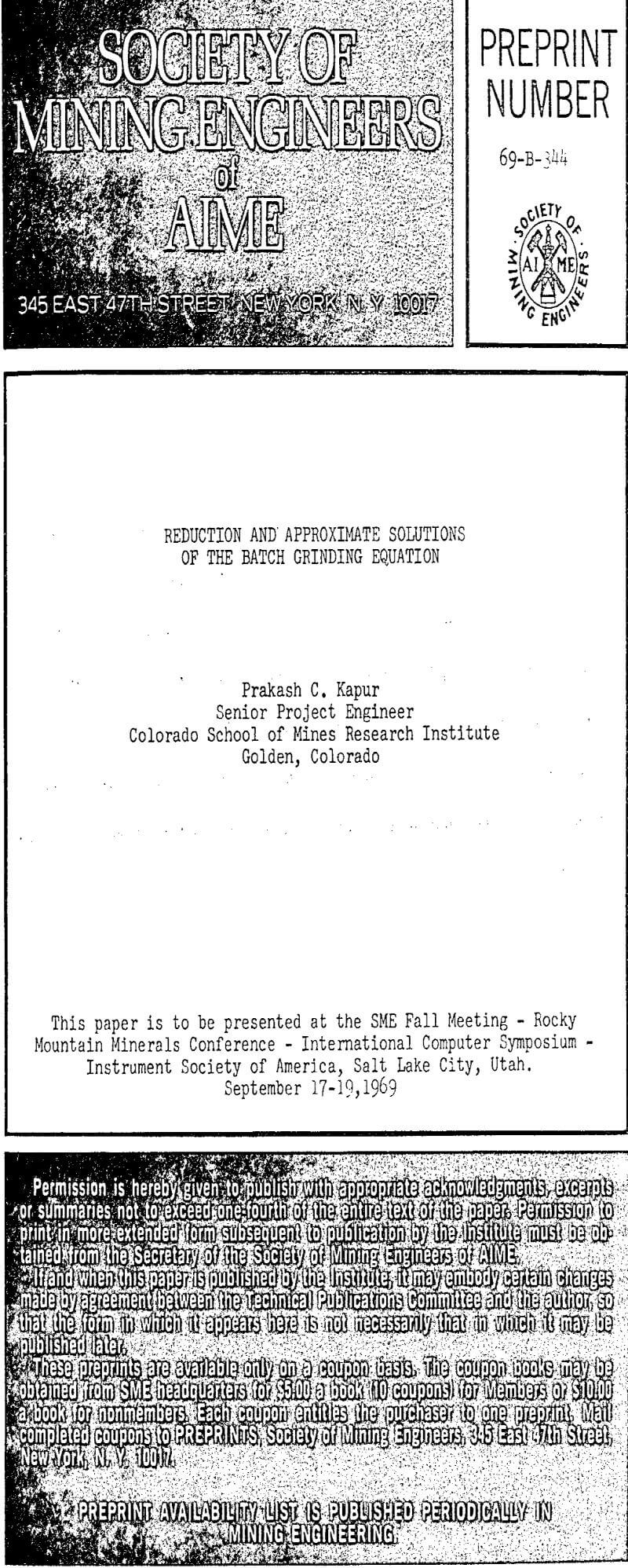By |2019-08-12T07:08:11-04:00July 23rd, 2019|Categories: Grinding|Tags: |Comments Off on Reduction and Approximate Solutions of the Batch Grinding Equation

## BUY Laboratory & Small Plant Process Equipment

We have all the laboratory and plant equipment you need to test or build/operate your plant.

### ENTER our Mining Equipment' Store

We Sell EQUIPMENT for all types of Mineral Treatment PROCESSES and Laboratory Testing needs

## Do you need METALLURGICAL TESTING of your ORE?

View the Services we Provide

### 911Metallurgist Mineral Processing & Process Development Laboratory

We have a metallurgical test for every possible mineral type and treatment.

## Need ENGINEERING Services or Plant TROUBLESHOOTING?

We can IMPROVE ALL PLANTS / Mineral Processing Engineering & LABORATORY Ore Testing

### 911Metallurgy Engineering

Contact us for process engineering, metallurgical investigations, plant optimization, plant troubleshooting, needs. WE “FIX” METALLURGY.

I Need Ore Laboratory Testing